# A large

A large gear will be used to turn a smaller gear. The large gear will make 75 revolutions per minute. The smaller gear must make 384 revolutions per minute. Find the smallest number of teeth each gear could have. [Hint: Use either GCF or LCM. ]

Correct result:

a =  128
b =  25

#### Solution:

$b=75\mathrm{/}k=75\mathrm{/}3=25$We would be pleased if you find an error in the word problem, spelling mistakes, or inaccuracies and send it to us. Thank you!Tips to related online calculators
Do you want to calculate least common multiple two or more numbers?
Do you want to calculate greatest common divisor two or more numbers?
Need help calculate sum, simplify or multiply fractions? Try our fraction calculator.
Check out our ratio calculator.
Do you want to convert velocity (speed) units?

#### You need to know the following knowledge to solve this word math problem:

We encourage you to watch this tutorial video on this math problem:

## Next similar math problems:

• An airplaneAn airplane flies 1440 km in 2 1/4 hours. What is its average speed in km per hour?
• The fastestThe fastest boat can reach speeds more than 710% as fast as the Queen Mary 2. How would you express this number as a fraction and as a decimal?
• Doug bikedDoug biked 5 1/4 miles in 3/4 of an hour. What is his average speed?
• Walking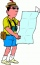Lucie can walk about 3 4/5 miles each hour. How far can she walk in 2 hours 45 minutes?
• Direct route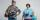From two different places A and B connected by a direct route, Adam (from city A) and Bohus (from city B) started at a constant speed. As Adam continued to go from A to B, Bohus turned around at the time of their meeting and at the same speed he returned
• Average speedWhen the bus stops at bus stops driving average speed is 45 km/h. If it did not stop it drive at speed 54 km/h. How many minutes of every hour it spend at stops?
• DoublingThe message is spreading that each day has doubled the number of people who know about it. All know message for 20 days. How long known it eighth people?
• Unit rateFind unit rate: 6,840 customers in 45 days
• PumpsPump that draws water at velocity 3.5 liters per second water from a construction trench take 35 minutes. a) Find out how many minutes the water would run out of the trench pump that draws 7.4 liters of water per second. b) What is the pumping velocity wo
• Typing course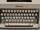Before taking a typing course, Terrence could type 39 words per minute. By the end of the course, he was able to type 68 words per minute. Find the percent increase.
• Car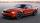The car goes from point A to point B at speed 86 km/h and back 53 km/h. If it goes there and back at speed 67 km/h trip would take 10 minutes shorter. What is the distance between points A and B?
• HandsThe clock shows 12 hours. After how many minutes will angle between the hour and minute hand 90°? Consider the continuous movement of both hands hours.
• TroopsThe route is long 147 km and the first-day first regiment went at an average speed of 12 km/h and journey back 21 km/h. The second day went second regiment the same route at an average speed of 22 km/h there and back. Which regiment will take route longer
• Motion problemFrom Levíc to Košíc go car at speed 81 km/h. From Košíc to Levíc go another car at speed 69 km/h. How many minutes before the meeting will be cars 27 km away?
• Motion2Cyclist started out of town at 19 km/h. After 0.7 hours car started behind him in the same direction and caught up with him for 23 minutes. How fast and how long went car from the city to caught cyclists?
• Washer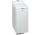The washing machine drum washes at 71 RPM. The washing machine motor pulley has a diameter of 8 cm. What must be the diameter of the drum machine pulley when the motor is at 351 RPM?
• Gear wheelsTwo gear wheels, which fit together, have the number of teeth z1=58 and z2=149. Calculate the speed of the first wheel, if the second wheel rotates 1232 revolutions per minute.